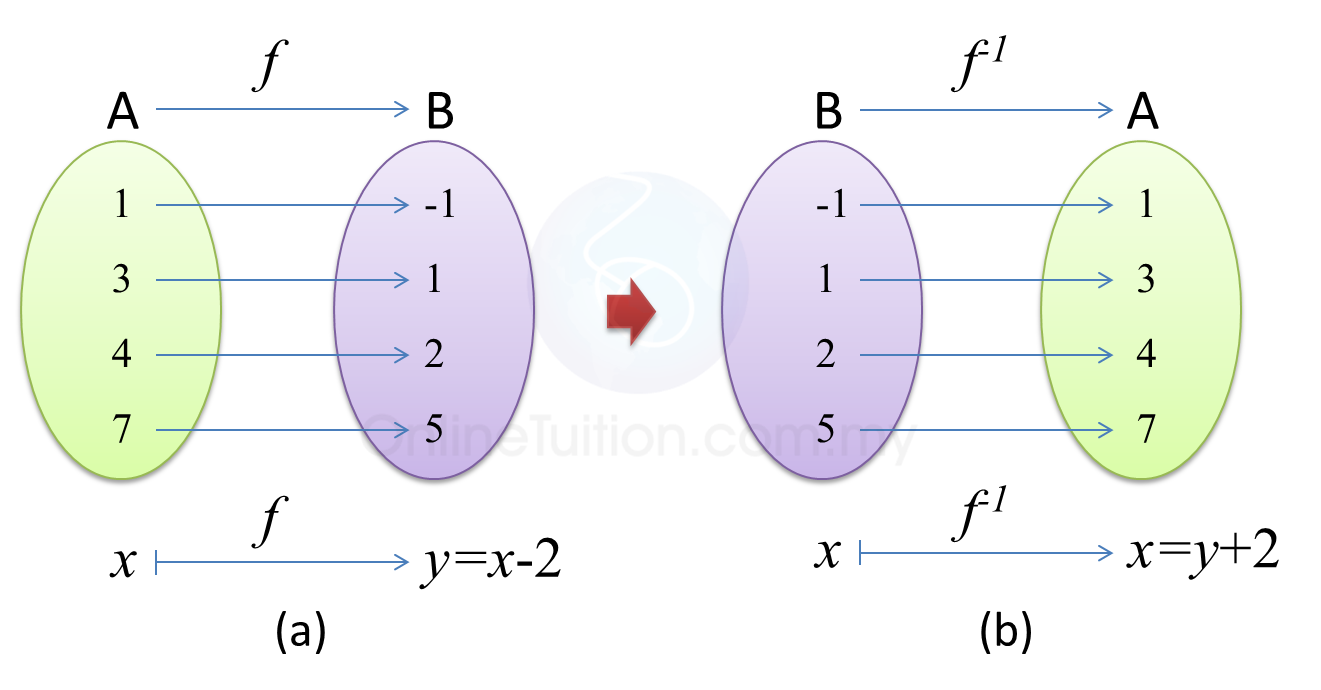1.5 Inverse Function

Inverse  Functions

1. Consider the function (f : x maps to  – 2) with domain A = {1, 3, 4, 7}. Then the range of the function is B = {-1, 1, 2, 5}. The arrow diagram representing this function is shown as below.2. If the arrows of (a) are reversed, the arrow diagram in (b) is obtained. A new function having domain B and range A is formed from the function f.  This new function is called the inverse function of f and is denoted by  f-1 .

3. To Find the inverse function, ${f}^{-1}\left(x\right)$ of $f\left(x\right)$
Put the function equal to y.
Rearrange to give x in term of y.
Rewrite as ${f}^{-1}\left(x\right)$  replacing y by x.

Example: Given $f\left(x\right)=5x-4$   , find the inverse function.